# Fatou theorem

(diff) ← Older revision | Latest revision (diff) | Newer revision → (diff)

in the theory of functions of a complex variable

Suppose that the harmonic function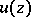,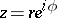, can be represented in the unit disc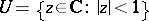by a Poisson–Stieltjes integralwhereis a Borel measure concentrated on the unit circle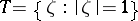,. Then almost-everywhere with respect to the Lebesgue measure on,has angular boundary values (cf. Angular boundary value).

This Fatou theorem can be generalized to harmonic functions,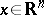,, that can be represented by a Poisson–Stieltjes integral in Lyapunov domains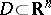(see , ). For Fatou's theorem for radial boundary values (cf. Radial boundary value) of multiharmonic functions in the polydisc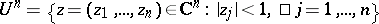see , .

Ifis a bounded analytic function in, then almost-everywhere with respect to the Lebesgue measure onit has angular boundary values.

This Fatou theorem can be generalized to functions of bounded characteristic (cf. Function of bounded characteristic) (see ). Points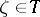at which there is an angular boundary value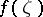are called Fatou points. Regarding generalizations of the Fatou theorem for analytic functionsof several complex variables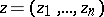,, see ; it turns out that forthere are also boundary values along complex tangent directions.

If the coefficients of a power series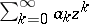with unit disc of convergencetend to zero,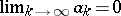, then this series converges uniformly on every arc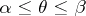of the circleconsisting only of regular boundary points for the sum of the series.

Ifand the series converges uniformly on an arc, it does not follow that the points of this arc are regular for the sum of the series.

Theorems 1), 2) and 3) were proved by P. Fatou .